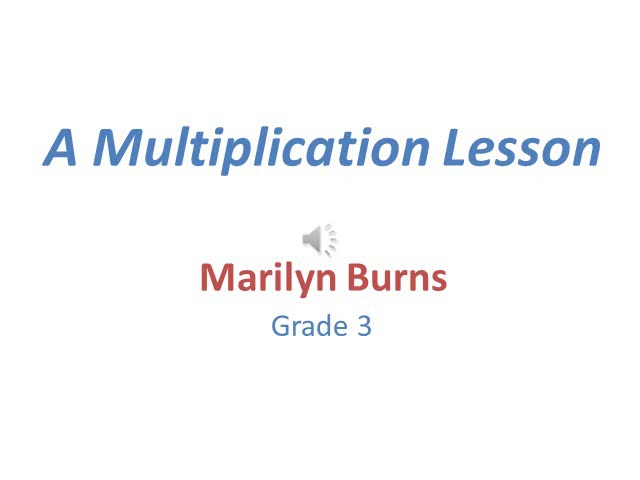# Word Problems: Don’t Put the Cart Before the Horse

by Math Solutions Professional Learning Team, September 16th, 2017All Blog Posts

A long-standing instructional practice has been to teach students how to add, subtract, multiply, and divide and then, after students have learned to compute, give them word problems to solve. I think of this instructional practice as putting the cart before the horse.

Instead of teaching computation first and then assigning word problems, word problems are effective vehicles for helping students develop understanding of the meanings of operations. Word problems build understanding of when and how operations are useful. This focus on understanding is important before students focus on learning and practicing procedures for calculating.

As an example, here I focus on multiplication and present “Multiplication Stories,” a lesson that I’ve taught many times to third graders. Also, in this post I’m experimenting with a format that’s new for me, a video that’s basically a Power Point presentation that I’ve narrated to describe how the lesson can unfold in a classroom. The video runs 7:36 minutes, and I’m interested in learning if this format is useful for helping communicate a teaching idea.

Word problems are effective when developing understanding of the meaning of multiplication, not after students learn to compute.

Here’s some background information. Before I teach the lesson in the video, I usually teach three other lessons to the class―”Things That Come in Groups,” “Circles and Stars” (I don’t know how I ever taught multiplication without this game), and a lesson based on the children’s book, Amanda Bean’s Amazing Dream. (These lessons and the one on the video all appear in Teaching Arithmetic: Lessons for Introducing Multiplication, Grade 3.)

Take a look. And then please let me know if you find a video like this useful for describing instruction. If so, I’m interested in hearing why and/or what suggestions you have for me to improve it.Note: This is the lesson that sparked an astonishing discovery from Lydia, a third grader, that I described in my previous post, Where’s the Math?